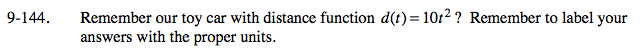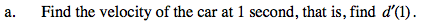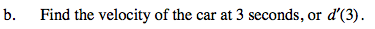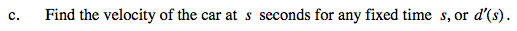### Home > PCT > Chapter Ch9 > Lesson 9.3.4 > Problem9-144

9-144.Find the slope function.

$d'(t)=\lim_{h\rightarrow 0}\frac{10(t+h)^{2}-10t^{2}}{h}$Substitute t = 1 into the slope function.Substitute t = 3 into the slope function.Substitute t = s into the slope function.